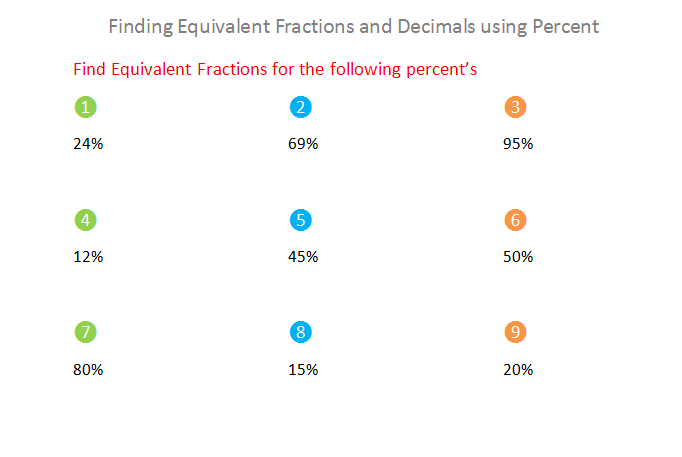## Preview

Finding equivalent fractions and decimals using percents is a really long title but actually the topic is really easy.
So first let's find an equivalent fraction using a percent and to do that we need a percent so let's take 22% and now we have to make the percent into a fraction like this:
22% = 22
100
And now we have to make an equivalent fraction so we have to multiply both the numerator and denominator with the same number so multiply them by the number 5 ( or any other number )like this:
22% = 22   =  22  x 5= 110
100     100 x 5= 500
So now we know that an equivalent fraction for the fraction 22/100 is 110/500.

Now let's find a decimal using a percent and to do that we have to take a percent so let's take the percent 45% and we have to make it into a fraction like this:
45% = 45
100
And now we have to make it into a decimal by dividing 45 by 100 like this:
45   = 0.45
100
So now you know that the decimal number for the fraction 45/100 is 0.45.
Now try some of these and make sure to watch the video on YouTube.Sheet 1 How to Find Equivalent Fractions